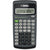## Texas Instruments TI-30XA Student Scientific Calculator

The Texas Instruments TI 30XA Scientific Calculator makes it easier for you to perform operations for math and science classes. It features a large, easy-to-read LCD. This student scientific calculator's slide cover protects the buttons and screen from damage caused by drops and impacts. It's suitable for use in most high school and middle school classes.  Texas Instruments TI-30XA Student Scientific Calculator: 10-digit display; for general math, pre-algebra, algebra 1 and 2, trigonometry and biology Performs trigonometric functions, logarithms, roots, powers, reciprocals, and factorials Also add, subtract, multiply and divide fractions; 1-variable statistics (mean/standard deviation) Conversions: fractions/decimals, degrees/radians/grads, DMS/decimal/degrees and polar/rectangular Texas Instruments scientific calculator is battery powered; includes slide case
\$12.00
Availability: 5 In Stock
Texas Instruments

The Texas Instruments TI 30XA Scientific Calculator makes it easier for you to perform operations for math and science classes. It features a large, easy-to-read LCD. This student scientific calculator's slide cover protects the buttons and screen from damage caused by drops and impacts. It's suitable for use in most high school and middle school classes.

Texas Instruments TI-30XA Student Scientific Calculator:

• 10-digit display; for general math, pre-algebra, algebra 1 and 2, trigonometry and biology
• Performs trigonometric functions, logarithms, roots, powers, reciprocals, and factorials
• Also add, subtract, multiply and divide fractions; 1-variable statistics (mean/standard deviation)